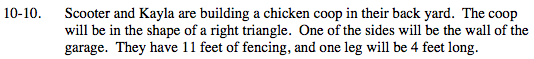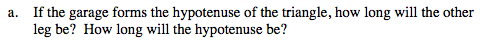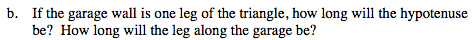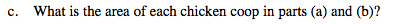### Home > MC2 > Chapter 10 > Lesson 10.1.1 > Problem10-10

10-10.If the garage forms the hypotenuse, then the fencing will make up the legs of the triangle.
If there is 11 feet of fencing, and one side of the triangle is 4 feet long, what is the length of the other leg?

The other leg will be 7 feet long. To find the length of the hypotenuse use the Pythagorean theorem.

42 + 72 = x2If the garage wall is a leg of the triangle instead of the hypotenuse, then its value cannot be greater than 7.
The side with a value of 7 is, therefore, the new hypotenuse.

The leg along the garage will be 5.74 ft.The new Pythagorean equation is: 42 + x2 = 72

To find each area, multiply the two legs (since they are perpendicular to each other and therefore form the base and the height) and divide that product by 2.

The area for (a) is 14 ft2.
What is the area for (b)?